MEMO 2009 ekipno problem 5

Kvaliteta:
Avg: 3,0
Težina:
Avg: 6,0
Let$ABCD$ be a parallelogram with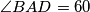$\angle BAD = 60$ and denote by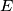$E$ the intersection of its diagonals. The circumcircle of triangle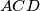$ACD$ meets the line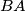$BA$ at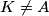$K \ne A$, the line$BD$ at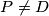$P \ne D$ and the line$BC$ at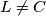$L\ne C$. The line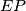$EP$ intersects the circumcircle of triangle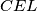$CEL$ at points$E$ and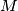$M$. Prove that triangles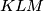$KLM$ and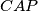$CAP$ are congruent.
Izvor: Srednjoeuropska matematička olimpijada 2009, ekipno natjecanje, problem 5